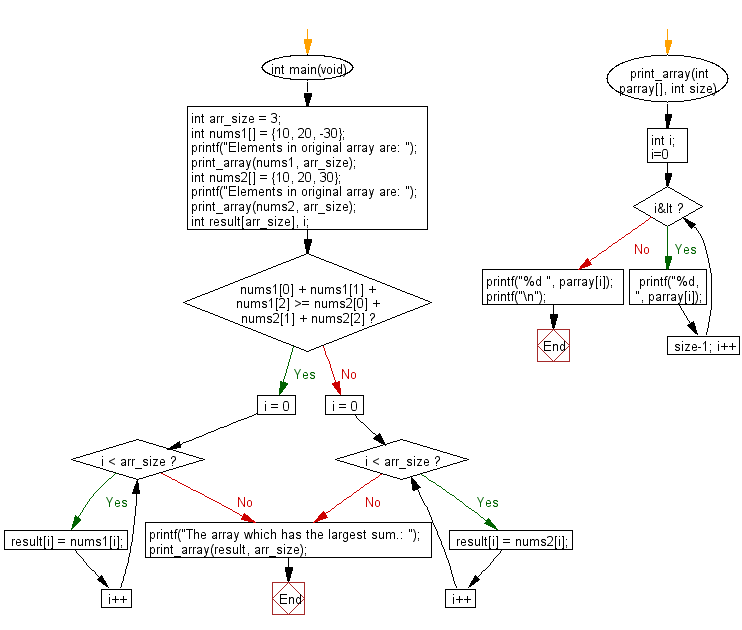﻿ C : Find the largest sum of two arrays of three integers# C Exercises: Compute the sum of the two given arrays of integers of length 3 and find the array which has the largest sum

## C-programming basic algorithm: Exercise-45 with Solution

Write a C program to compute the sum of the two given arrays of integers of length 3 and find the array which has the largest sum.

C Code:

``````#include <stdio.h>
#include <stdlib.h>
int main(void){
int arr_size = 3;
int nums1[] = {10, 20, -30};
printf("Elements in original array are: ");
print_array(nums1, arr_size);
int nums2[] = {10, 20, 30};
printf("Elements in original array are: ");
print_array(nums2, arr_size);
int result[arr_size], i;
if ( nums1 + nums1 + nums1 >= nums2 + nums2 + nums2)
{
for (i = 0; i < arr_size; i++) {
result[i] = nums1[i];
}
}
else
{
for (i = 0; i < arr_size; i++) {
result[i] = nums2[i];
}
}
printf("The array which has the largest sum.: ");
print_array(result, arr_size);
}
print_array(int parray[], int size)
{
int i;
for( i=0; i<size-1; i++)
{
printf("%d, ", parray[i]);
}
printf("%d ", parray[i]);
printf("\n");
}
``````

Sample Output:

```Elements in original array are: 10, 20, -30
Elements in original array are: 10, 20, 30
The array which has the largest sum.: 10, 20, 30
```

Pictorial Presentation:Flowchart:C Programming Code Editor:

What is the difficulty level of this exercise?

Test your Programming skills with w3resource's quiz.

﻿

## C Programming: Tips of the Day

Why doesn't a+++++b work?

printf("%d",a+++++b); is interpreted as (a++)++ + b according to the Maximal Munch Rule!.

++ (postfix) doesn't evaluate to an lvalue but it requires its operand to be an lvalue.

! 6.4/4 says the next preprocessing token is the longest sequence of characters that could constitute a preprocessing token"

Ref : https://bit.ly/3fdldUT